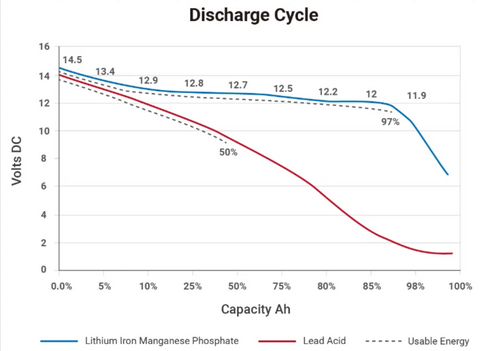# How to Measure Battery Capacity

If your electric boat battery drains faster than it should or faster than you need, then measuring its capacity is a necessary step. This blog will teach you how to correctly measure the battery capacity so that your future energy carriers work as expected and create no troubles for your engine - electric outboard motor, trolling motors or other variations of electric propulsion.

## Battery Capacity

Battery capacity is the total amount of electricity that the battery produces as a result of electrochemical reactions in it.

The capacity may change according to its electrolyte's structure, size, age, and density. Remember that excessive heat causes wear on lead grates for lead acid batteries.

The charge and discharge curves of batteries are exponential, not linear, as seen in the graphic below.As shown in the graphic, the higher the battery discharge, the lower the battery capacity. Peukert law states that a battery's capacity is determined by dividing its total Ah by its current value. For example, if you have a fully charged 100Ah battery that supplies 1C (5A) current, that battery will last 20 hours.

The actual battery capacity is usually measured in Watt-Hours (Wh). Such a method considers the voltage variation as the battery discharges. Ampere Hour (Ah) is often used as a proxy for capacity when voltage is assumed to be within the usable range and the exact voltage at which current is delivered over time is unimportant.

## Milliamp-Hours Capacity

The most quick-and-easy way to measure your battery capacity is to connect it to a constant current load, then count the time it takes to discharge the battery. Some special purpose battery charges also have a discharge function that lets you check the mAh(milliamp hour) capacity. Use professional equipment to measure these values accurately.

Remember that the measured mAh depends on the voltage you are willing to discharge the battery. Discharging the battery low, for example, to 2.8V every time may reduce its life. Besides, your circuit may require around 3.6V to function, so you may be unable to use the battery's total capacity as rated by the manufacturer.

## Watt-Hours Capacity

Another way to measure the battery's capacity is Watt-hours (Wh). Watt-hours is the number of Amps multiplied by the battery voltage. For example, a 12V100 (a 12-volt battery with a capacity of 100Ah) has a total of 12 x 100 = 1200Wh.

## Lithium Battery Capacity

For lithium batteries, we recommend you use Watt Hour (Wh). The main benefit is that you could quickly and precisely calculate the runtime without considering the voltage. You divide the capacity with the power, and you will get the runtime, which is very simple. For example, Spirit 1.0 Plus battery is 1276 Wh, and the full-throttle power is 1000 W. The runtime is 1.276 hours (1276 Wh / 1000 W), which is 1 hour 15 min. If you're looking for good-quality, durable batteries, take a look at Lithium Batteries. Their Lithium ion cells are a perfect addition to electric motor brands such as ThrustMe, TEMO, and ePropulsion.

## Methods to Measure Battery Capacity

• Constant current

Hook up the battery to a constant current load and measure how long it takes the battery to drain to a specified voltage. Capacity in Ah = Current in Amperes* Time in Hours

• Constant power

Same as above, but use a continuous power load

Capacity in Wh = Power in Watts * Time in hours

• Constant resistance

The constant resistance method requires an elementary discharge circuit - a single resistor. Connect the resistor across the battery terminals and let the battery discharge to a specific minimum voltage. Measure the voltage across the resistor. Take readings as frequently as possible. Power = V^2/R and Energy = Power * Time (where time is the length of your measurement interval). So you can calculate the energy for each measurement cycle. Add measurements for all processes to get total capacity in Wh.